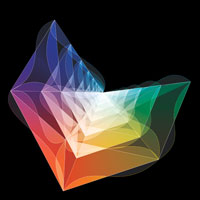Quantum Gravity Edited by Andy Ross Physicists are searching for a theory of quantum gravity. Most physicists believe that gravitons give rise to gravity. But calculations of graviton interactions yield infinities.Supergravity posits the existence of new particles that mirror graviton effects. It has long been assumed to suffer from the infinity problem, but no one was sure. Using new tools, UCLA theorist Zvi Bern and his team are now calculating these gravitational interactions and making sense.General relativity describes gravity perfectly on the macroscopic scale but goes wrong for gravitons. Feynman diagrams represent the scattering amplitudes for all the possible particle interactions, and physicists sum them to calculate the probabilities. Each new level of precision requires drawing exponentially more diagrams. For some particle interactions infinities cancel, but for gravitons they don't. Supergravity adds new symmetries to the theory. If one type of particle exists, then so does its opposite. The N = 8 supergravity variant has eight such doublings, and the mirror-image particles let physicists cancel some possibilities. This approach works for 4-loop precision. But experts suspect infinities reappear for more loops.Years ago, Bern and colleagues developed techniques for speeding up scattering amplitude calculations. Applying the techniques to supergravity calculations, they saw that gravitons behave like two copies of gluons, the strong nuclear force bosons in quantum chromodynamics. The success of QCD suggests that supergravity might work.Bern and his collaborators will test N = 8 supergravity by calculating what happens when gravitons collide to 5-loop precision in a fictional world with 4.8 spacetime dimensions. The 5-loop calculation for 4.8D roughly corresponds to a much more difficult 7-loop calculation in 4D. Black holes involve particles colliding with the Planck energy. At such high energies, an infinite number of Feynman diagrams are needed to make even a rough approximation of the scattering amplitude. This cripples calculations in N = 8 supergravity and suggests information is lost, which is a problem. To solve it, physicists suspect they will ultimately have to give up locality.Abandoning locality could mean abandoning Feynman diagrams, which assume locality. In one approach to calculating scattering amplitudes in supersymmetric quantum physics, gluon scattering amplitudes are computed by measuring the volume of an amplituhedron, a geometric object whose shape is determined by the number and properties of the gluons involved in an interaction. The approach does not assume locality.The amplituhedron corresponds to interactions between gluons, and so the fact that gravitons behave like two copies of gluons could point the way forward. If the latest supergravity calculation of graviton scattering in 4.8D works, then N = 8 supergravity may be a viable theory. If not, something is missing. Many are looking to string theory.The Amplituhedron Edited by Andy Ross Quantum field theory would be simpler if interactions previously calculated with long formulas matched the volume of the corresponding amplituhedron. A geometric version of QFT could also help in the search for a theory of quantum gravity by removing locality and unitarity. Locality is the notion that particles can interact only when contiguous in spacetime. Unitarity says the probabilities of all possible outcomes of an interaction add up to one. The concepts are central to QFT.The new geometric approach to particle interactions avoids assuming locality and unitarity, which break down in quantum gravity. If spacetime and probabilities emerge from the geometry, a geometric object related to the amplituhedron might describe gravity and show why particles appear to move in 4D spacetime.The amplituhedron encodes scattering amplitudes representing the probability that a certain set of particles will turn into certain other particles upon colliding. The volume of a master amplituhedron with an infinite number of facets represents the total amplitude of all physical processes. Lower-dimensional amplituhedra in the master structure correspond to interactions between finite numbers of particles.String theory is a candidate theory of quantum gravity. A duality exists between string theory and QFT in a different number of dimensions. The amplituhedron research could show how our universe emerges out of pure geometry.